NCERT Solutions for Class 7 Maths Chapter 2 Fractions and Decimals Ex 2.1 are part of NCERT Solutions for Class 7 Maths. Here we have given NCERT Solutions for Class 7 Maths Chapter 2 Fractions and Decimals Ex 2.1.

 Board CBSE Textbook NCERT Class Class 7 Subject Maths Chapter Chapter 2 Chapter Name Fractions and Decimals Exercise Ex 2.1 Number of Questions Solved 8 Category NCERT Solutions

## NCERT Solutions for Class 7 Maths Chapter 2 Fractions and Decimals Ex 2.1

Question 1.
Solve :Solution: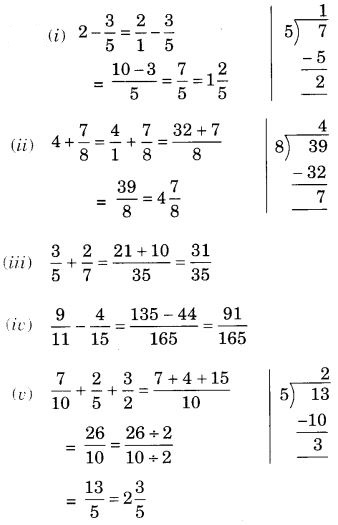Question 2.
Arrange the following in descending order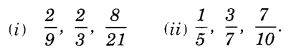Solution:
(i) Converting the given fractions into like fractions, we have(ii) Converting the given fractions into like fractions, we have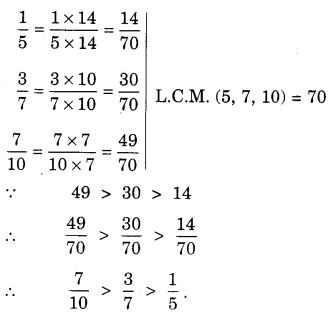Question 3.
In a “magic square”, the sum of the numbers in each row, in each column and along the diagonals is the same. Is this a magic square?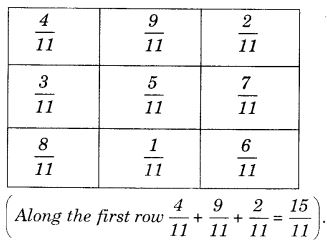Solution:
Yes ! this is a magic square.

Question 4.
A rectangular sheet of paper is 12 $$\frac { 1 }{ 2 }$$ cm long 10 $$\frac { 2 }{ 3 }$$ cm wide. find its perimeter
Solution:
Perimeter of the rectangular sheet of paperQuestion 5.
Find the perimeters of (i) A ABE (ii) the rectangle BCDE in this figure. Whose perimeter is greater?
Solution:
(i) Perimeter of ∆ ABE = AB + BE + EA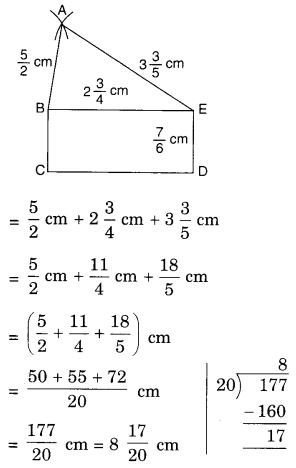(ii) Perimeter of the rectangle BCDE = BC + CD + DE + EB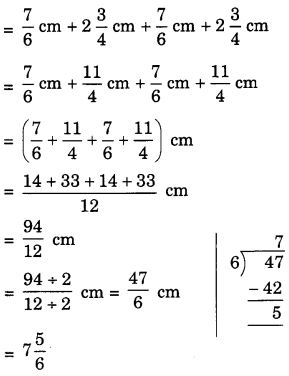So, the perimeter of A ABE is greater than the perimeter of the rectangle BCDE.

Question 6.
Salil wants to put a picture in a frame. The picture is 7 $$\frac { 3 }{ 5 }$$ cm wide. To fit in the frame the picture cannot be more than 7 $$\frac { 3 }{ 10 }$$ cm wide. How much should the picture be trimmed?
Solution:
The picture should be trimmed byQuestion 7.
Ritu are $$\frac { 3 }{ 5 }$$ part of an apple and the remaining apple was eaten by her brother Somu. How much part of the apple did Somu eat? Who had the larger share? By how much?
Solution:
Part of the apple ate by Somu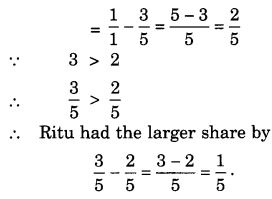Question 8.
Michael finished colouring a picture in $$\frac { 7 }{ 12 }$$ hour. Vaibhav finished colouring the same picture in $$\frac { 3 }{ 4 }$$ hour. Who worked longer? By what fraction was it longer?
Solution:We hope the NCERT Solutions for Class 7 Maths Chapter 2 Fractions and Decimals Ex 2.1 help you. If you have any query regarding NCERT Solutions for Class 7 Maths Chapter 2 Fractions and Decimals Ex 2.1, drop a comment below and we will get back to you at the earliest.